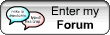| HISTORY | PHILOSOPHY | RELIGION | SCIENCE | ECONOMY |
| SYNCHRONY |GENEALOGICAL TABLES | HISTORICAL MAPS |
| ITALIAN VERSION |

1300 B.C.

THE THEOREM OF PYTHAGORAS

In the tablet, of which the translation is reported, it seems really that the theorem of Pythagoras is applied.

In fact the calculation of the sides of a rectangle is exactly performed beginning from the knowledge of the diagonal (0,6666) and of the relationship existing between the width (W) and the length (L): W=L-L/4.

Place: Susa (Mesopotamia)

Epoch: 1300 b.C. - End of the I Dynasty of Babylon

Tablet of Susa

Problem

We set that:

- the width (of the rectangle) measures a quarter less in relationship to the length.

Width = Length - Length/4

- the dimension of the diagonal is 0,6666.

Diagonal = 0,6666

Which are the length and the width of the rectangle?

Solution

Set 1, the length, set 1 the prolongation.

Arbitrary length = 1

0,25, the quarter, subtract from 1, you find 0,75.

Arbitrary width = 1 - 0,25 = 0,75

Set 1 as length, set 0,75 as width, square 1, the length, you find 1.

1^1 = 1

Square 0,75, the width, you find 0,5625.

0,75^1 = 0,5625

Of 1 and 0,5625 make the sum: 1,5625.

1+ 0,5625 = 1,5625

Which is the square root? The square root is 1,25.

1,5625^0,5 = 1,25

Considering that 0,6666 is the diagonal that has been indicated to you, solve the inverse of 1,25 the diagonal, you find 0,8. Bring 0,8 to 0,6666 the diagonal that has been said to you, you find 0,5333.

0,6666/1,25 = 0,5333

Result

Bring 0,5333 to 1 the length that you have set: you find 0,5333, the length is 0,5333.

0,5333*1 = 0,5333

Bring 0,5333 to 0,75 the width that you have set: you find 0,4. It is the width.

0,5333*0,75 = 0,4

Bibliographical references:

 Pichot A. La nascita della scienza: Mesopotamia - Egitto - Grecia Dedalo

MAAT FORUMCONOSCERE LA STORIA PER CREARE IL FUTURO
TO KNOW THE HISTORY TO CREATE THE FUTURE